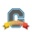cancel
Showing results for
Did you mean:
Surveyor

## Points vs Points?

I've written an assignment in Quizzes.Next, it is worth 16 points and will not be counted towards a final grade in the Gradebook (so the assignment group it is in is 0% weighting). I've set it so the score will be reported as a %.

I've added this assignment to a module in the course but the subheading under the assignment title reads 0 points.I can see where I could change this (click on the triple dots at the end and Edit) but is there any need?

When a student submits the assignment (and let's say gets 12/16) will it is display as 75% in the gradebook or 0?

I'm not sure what is the difference between the total points of the questions in the quiz (16) and points displayed as above in the module (0) and why they are different.

Many thanks

Tags (3)Community Coach

Hi fiona.wilson999@btinternet.com .  This is a good question and there are many possible variations between the two places where points can be determined in Quizzes.Next, setting points v. percent, and being able to place the assignment in a group with or without weight.  I thought this was interesting so did some testing in general and here is what I found, although it would be interesting to see if others notice the same.

If the assignment is set to points, and there is a difference between the point value of the assessment overall and the total value of all questions, when completed the student will be shown what percent they received based on their score on the quiz.  The point value in the gradebook appears to be based on calculating their percent on the assessment multiplied by the total point value of the quiz set in the general quiz settings.  However, if the assignment is set to percentage instead of point, the percent displayed in the gradebook seems to be the same as the students are shown (points earned/points possible on the quiz) as opposed to points earned/points for the assessment given in the general quiz settings.  I'm sure that behind the scenes, the final grade is calculating points earned from the general quiz setting as opposed to the total value of all of the question points though.

I did test adding the quiz to an assignment group that is weighted or not weighted into the final grade.  If the assignment is in an unweighted group, it doesn't seem to appear in the teacher gradebook at all.  One can go into each student and see what percent they received on the quiz and the point value of the assignment in general, but it indicates that the assignment is in a group worth zero percent of the final grade.  When in a group weighted for the final grade, the assignment shows in the gradebook with other graded assignments.

I'm going to try and do more testing, but it seems as though depending on the faculty settings, it would be good to keep in mind how the two point value locations in Quizzes.Next function.

I hope this helps (and makes sense)!